# Strong Stieltjes moment problem

The strong Stieltjes moment problem for a given sequence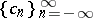of real numbers is concerned with finding real-valued, bounded, monotone non-decreasing functionswith infinitely many points of increase forsuch that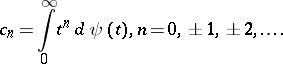(a1)

This problem, which generalizes the classical Stieltjes moment problem (where the given sequence is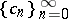; cf. also Krein condition), was first studied by W.B. Jones, W.J. Thron and H. Waadeland [a1].

Let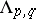be the complex linear space spanned by the set of functionswith, and define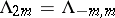and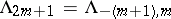for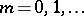, and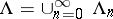. An element ofis called a Laurent polynomial. For a given sequence, a necessary and sufficient condition for the strong Stieltjes moment problem to be solvable is that the linear operatordefined on the base elements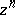of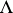by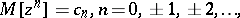(a2)

is positive on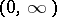, i.e. for anysuch that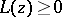for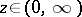and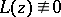, then. An equivalent condition is that if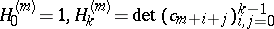(a3)

for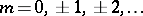,, are the Hankel determinants associated with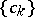(cf. also Hankel matrix), thenOrthogonal Laurent polynomials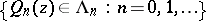may be defined with respect to the inner product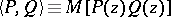and are given by:(a4)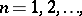and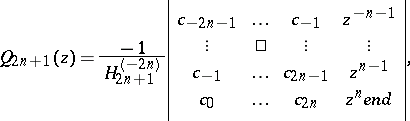(a5)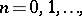and. Corresponding associated orthogonal Laurent polynomials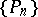are defined by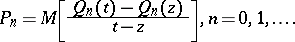(a6)

The rational functionsare the convergents of the positive T-fraction [a3],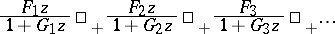(a7)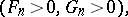where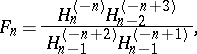which corresponds to the formal pair of power series,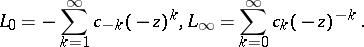(a8)

The T-fraction is equivalent to the continued fraction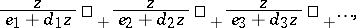(a9)

where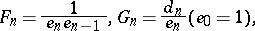(a10)The following result may then be proved [a1]: The solution of the strong Stieltjes moment problem (a1) is unique if and only if at least one of the series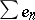,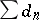diverges, and then(a11)

where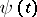is this unique solution.The convergence is uniform on every compact subset of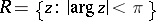.

The strong Stieltjes moment problem is said to be determinate when it has a unique solution and indeterminate otherwise. A detailed discussion of the latter case has been given in [a4].

A classic example of a strong Stieltjes moment problem is the log-normal distribution,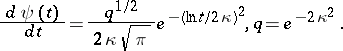(Cf. also Normal distribution.) The corresponding sequence of moments is, where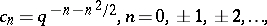and the strong Stieltjes moment problem in this case is indeterminate [a5]. The moments corresponding to the log-normal distribution are related to a subclass of strong Stieltjes moment problems whereThis subclass has been called strong symmetric Stieltjes moment problems by A.K. Common and J. McCabe, who studied properties of the related continued fractions [a6]. Other subclasses have been investigated in [a7].

Cf. also Moment problem.